Water Resources of the United States

The following documentation was taken from:

U.S. Geological Survey Water-Resources Investigations Report 94-4002: Nationwide summary of U.S. Geological Survey regional regression equations for estimating magnitude and frequency of floods for ungaged sites, 1993

# TEXAS

## HOUSTON URBAN

### Summary

Regression equations developed for urban streams in Houston are for estimating peak discharges (QT) having recurrence intervals T that range from 2 to 500 years. The explanatory basin variables used in the equations are drainage area (A), in square miles; the degree of urban development (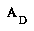), defined as the percentage of total contributing area within 200 feet of streets, roads, parking lots, and industrial sites that is drained by open street ditches or storm sewers; and the bankfull channel conveyance (K), in cubic feet per second, at the controlling section where K = (1.49/n) AC R2/3 from Mannings equation for open-channel flow (n = Mannings roughness coefficient, AC = channel cross-sectional area at controlling section, in square feet, and R = hydraulic radius, in feet). The drainage area (A) can be measured from topographic maps; the percentage of urban development () can be obtained from aerial photographs, or field reconnaissance; but bankfull channel conveyance computations will require a field survey. The regression equations were developed from peak-discharge records for 22 stations and are applicable to unregulated streams with drainage areas ranging from 1.33 to 182 square miles, bankfull channel conveyance ranging from 12,000 to 2,800,000 cubic feet per second, and percentage of urban development ranging from 37 to 99. The standard errors of estimate of the regression equations range from 17 to 25 percent.

### Procedure

Topographic maps, aerial photographs, field surveys, and the following equations are used to estimate the needed peak discharges QT, in cubic feet per second, having selected recurrence intervals T. Equations are of the following form: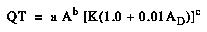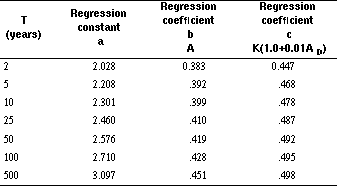### Reference

Liscum, F., and Massey, B.C., 1980, Technique for estimating the magnitude and frequency of floods in the Houston, Texas, metropolitan area: U.S. Geological Survey Water-Resources Investigations Report 80-17, 29 p.

## AUSTIN URBAN

### Summary

Regression equations developed for urban streams in Austin are for estimating peak discharges (QT) having recurrence intervals T that range from 2 to 100 years. The explanatory basin variables used in the equations are contributing drainage area (CDA), in square miles, and total percentage of drainage area that is impervious (TIMP). The variable CDA can be measured using topographic maps; TIMP can be estimated from aerial photographs or land-use maps. The regression equations were developed from simulated and recorded peak-discharge records at 13 sites on 7 streams, and the results are applicable to unregulated streams (not regulated by flood-control structures) having drainage areas between 2 and 20 square miles. The standard errors of estimate of the regression equations range from 26 to 30 percent.

### Procedure

Topographic maps, aerial photographs or land-use maps, and the following equations are used to estimate the needed peak discharges QT, in cubic feet per second, having selected recurrence intervals T.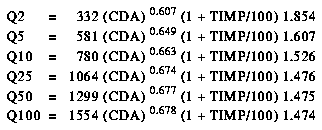### Reference

Veenhuis, J.E., and Gannett, D.G., 1986, The effects of urbanization on floods in the Austin metropolitan area, Texas: U.S. Geological Survey Water-Resources Investigations Report 86-4069, 66 p.

## DALLAS-FORT WORTH URBAN

### Summary

Regression equations developed for urban streams in Dallas-Fort Worth are for estimating peak discharges (QT) having recurrence intervals (T) that range from 2 to 100 years. The explanatory basin variables used in the equations are drainage area (DA), in square miles, and an urbanization index (UI), which is evaluated as described in the report by Land and others (1982). The regression equations were developed from peak-discharge records from drainage areas in the Dallas-Fort Worth area ranging from 1.25 to 66.4 square miles with results considered applicable to drainage areas between 3 and 40 square miles having urbanization indexes between 12 and 33. The standard errors of estimate of the regression equations are about 30 percent.

### Procedure

Topographic maps and the following equations are used to estimate the needed peak discharges QT, in cubic feet per second, having selected recurrence intervals T.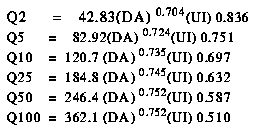### Reference

Land, L.F., Schroeder, E.E., and Hampton, B.B., 1982, Techniques for estimating the magnitude and frequency of floods in the Dallas-Fort Worth metropolitan area, Texas: U.S. Geological Survey Water-Resources Investigations Report 82-18, 55 p.

Figure 1. Flood-frequency region map for Texas. (PostScript file of Figure 1.)

Figure 2. Mean annual precipitation in Texas. (PostScript file of Figure 2.)